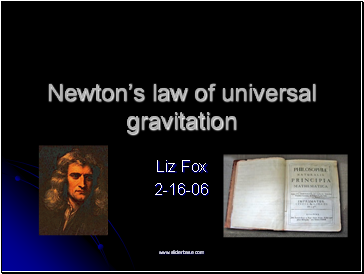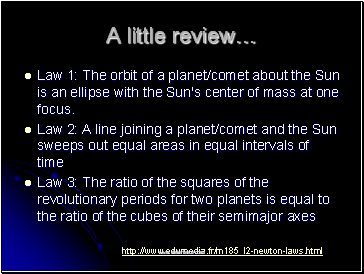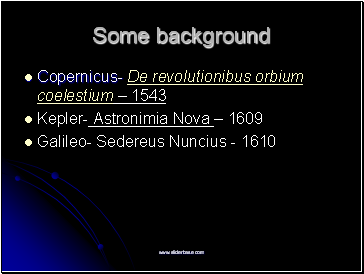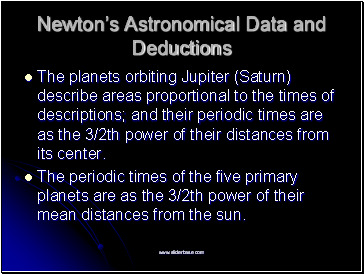# Newton’s law of universal gravitationPage 1

#### WATCH ALL SLIDES

Slide 1## Newton’s law of universal gravitation

Liz Fox

2-16-06

Slide 2A little review…

Law 1: The orbit of a planet/comet about the Sun is an ellipse with the Sun's center of mass at one focus.

Law 2: A line joining a planet/comet and the Sun sweeps out equal areas in equal intervals of time

Law 3: The ratio of the squares of the revolutionary periods for two planets is equal to the ratio of the cubes of their semimajor axes

Slide 3Some background

Copernicus- De revolutionibus orbium coelestium – 1543

Kepler- Astronimia Nova – 1609

Galileo- Sedereus Nuncius - 1610

Slide 4## Newton’s Principia

Mathematical Principles of Natural Philosophy

Published in 1687

Uses Kepler’s Laws to prove elliptical orbits

Explains behavior of tides, precession of the equinoxes, and the irregularities in the moon’s orbit

Slide 5## Newton’s Astronomical Data and Deductions

The planets orbiting Jupiter (Saturn) describe areas proportional to the times of descriptions; and their periodic times are as the 3/2th power of their distances from its center.

The periodic times of the five primary planets are as the 3/2th power of their mean distances from the sun.

Slide 6## “The nature of the forces”

The forces by which the primary planets are continually drawn off from rectilinear motions, and retained in their proper orbits, tend to the sun; and are inversely as the squares of the distances of the places of those planets from the sun’s center.

Slide 7## An Inverse-Square Law

Centripetal vs. centrifugal

Huygens- Horologium Oscillatorium (On Pendulum Clocks) - 1673

When 2 identical bodies move with the same velocity on unequal circumferences, their [centripetal] forces are in the inverse proportion to their diameters

When identical bodies move on unequal circumferences with unequal velocities the [centripetal] force of the faster is to that of the slower as the square of their velocities

Slide 8## Newton’s take

The centripetal forces of bodies tend to the centers of the same circles; and are to each other as the squares of the arcs described in equal times divided respectively by the radii of the circles.

Slide 9

Go to page:
1  2